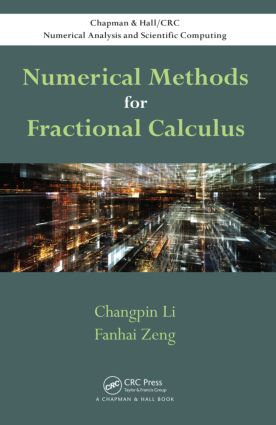Numerical Methods for Fractional Calculus

1st Edition

Chapman and Hall/CRC

300 pages | 12 B/W Illus.

Hardback: 9781482253801
pub: 2015-05-19
Currently out of stock
\$120.00
x
eBook (VitalSource) : 9780429075636
pub: 2015-05-19
from \$28.98

FREE Standard Shipping!

Description

Numerical Methods for Fractional Calculus presents numerical methods for fractional integrals and fractional derivatives, finite difference methods for fractional ordinary differential equations (FODEs) and fractional partial differential equations (FPDEs), and finite element methods for FPDEs.

The book introduces the basic definitions and properties of fractional integrals and derivatives before covering numerical methods for fractional integrals and derivatives. It then discusses finite difference methods for both FODEs and FPDEs, including the Euler and linear multistep methods. The final chapter shows how to solve FPDEs by using the finite element method.

This book provides efficient and reliable numerical methods for solving fractional calculus problems. It offers a primer for readers to further develop cutting-edge research in numerical fractional calculus. MATLAB® functions are available on the book’s CRC Press web page.

Reviews

"The book provides a survey of many different methods for the numerical computation of Riemann–Liouville integrals of fractional order and of fractional derivatives of Riemann–Liouville, Caputo, and Weyl type. Algorithms for the solution of associated ordinary differential equations and certain special classes of partial differential equations are presented as well."

Zentralblatt MATH 1326

Introduction to Fractional Calculus

Fractional Integrals and Derivatives

Some Other Properties of Fractional Derivatives

Some Other Fractional Derivatives and Extensions

Physical Meanings

Fractional Initial and Boundary Problems

Numerical Methods for Fractional Integral and Derivatives

Approximations to Fractional Integrals

Approximations to Riemann–Liouville Derivatives

Approximations to Caputo Derivatives

Approximation to Riesz Derivatives

Matrix Approach

Short Memory Principle

Other Approaches

Numerical Methods for Fractional Ordinary Differential Equations

Introduction

Direct Methods

Integration Methods

Fractional Linear Multistep Methods

Finite Difference Methods for Fractional Partial Differential Equations

Introduction

One-Dimensional Time-Fractional Equations

One-Dimensional Space-Fractional Differential Equations

One-Dimensional Time-Space Fractional Differential Equations

Fractional Differential Equations in Two Space Dimensions

Galerkin Finite Element Methods for Fractional Partial Differential Equations

Mathematical Preliminaries

Galerkin FEM for Stationary Fractional Advection Dispersion Equation

Galerkin FEM for Space-Fractional Diffusion Equation

Galerkin FEM for Time-Fractional Differential Equations

Galerkin FEM for Time-Space Fractional Differential Equations

Bibliography

Index

Changpin Li is a full professor at Shanghai University. He earned his Ph.D. in computational mathematics from Shanghai University. Dr. Li’s main research interests include numerical methods and computations for FPDEs and fractional dynamics. He was awarded the Riemann–Liouville Award for Best FDA Paper (theory) in 2012. He is on the editorial board of several journals, including Fractional Calculus and Applied Analysis, International Journal of Bifurcation and Chaos, and International Journal of Computer Mathematics.

Fanhai Zeng is visiting Brown University as a postdoc fellow. He earned his Ph.D. in computational mathematics from Shanghai University. Dr. Zeng’s research interests include numerical methods and computations for FPDEs.